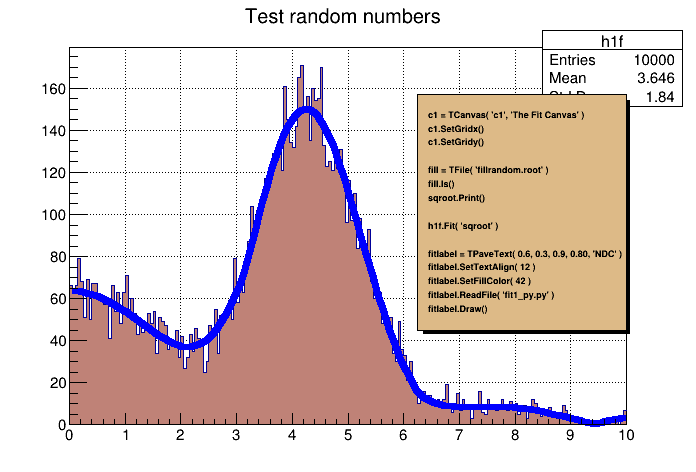# Fit 1¶

Fit example.

Author: Wim Lavrijsen
This notebook tutorial was automatically generated with ROOTBOOK-izer from the macro found in the ROOT repository on Thursday, June 04, 2020 at 02:44 AM.

In :
from os import path
from ROOT import TCanvas, TFile, TPaveText
from ROOT import gROOT, gBenchmark

c1 = TCanvas( 'c1', 'The Fit Canvas', 200, 10, 700, 500 )
c1.SetGridx()
c1.SetGridy()
c1.GetFrame().SetFillColor( 21 )
c1.GetFrame().SetBorderMode(-1 )
c1.GetFrame().SetBorderSize( 5 )

gBenchmark.Start( 'fit1' )

Welcome to JupyROOT 6.23/01


We connect the ROOT file generated in a previous tutorial

In :
fill = TFile( 'py-fillrandom.root' )


The function "ls()" lists the directory contents of this file

In :
fill.ls()

TFile**		py-fillrandom.root
TFile*		py-fillrandom.root
KEY: TFormula	form1;1	abs(sin(x)/x)
KEY: TF1	sqroot;1	x*gaus(0) + *form1
KEY: TH1F	h1f;1	Test random numbers


Get object "sqroot" from the file.

In :
sqroot = gROOT.FindObject( 'sqroot' )
sqroot.Print()

Formula based function:     sqroot
sqroot : x*gaus(0) + *form1 Ndim= 1, Npar= 4, Number= 0
Formula expression:
x*[p0]*exp(-0.5*((x-[p1])/[p2])*((x-[p1])/[p2]))+[p3]*(abs(sin(x)/x))


Now fit histogram h1f with the function sqroot

In :
h1f = gROOT.FindObject( 'h1f' )
h1f.SetFillColor( 45 )
h1f.Fit( 'sqroot' )

Out:
<cppyy.gbl.TFitResultPtr object at 0x5650b9e71a50>
 FCN=198.935 FROM MIGRAD    STATUS=CONVERGED     148 CALLS         149 TOTAL
EDM=2.98567e-07    STRATEGY= 1      ERROR MATRIX ACCURATE
EXT PARAMETER                                   STEP         FIRST
NO.   NAME      VALUE            ERROR          SIZE      DERIVATIVE
1  p0           3.31658e+01   5.45703e-01   3.00376e-03  -1.11540e-03
2  p1           4.00667e+00   1.65304e-02   9.48491e-05  -3.06425e-02
3  p2           9.84663e-01   1.28238e-02   6.05976e-05  -3.04244e-02
4  p3           6.34464e+01   1.33233e+00   8.77483e-03  -3.96109e-04

Error in <TFitResultPtr>: TFitResult is empty - use the fit option S
Error in <TFitResultPtr>: TFitResult is empty - use the fit option S
Error in <TFitResultPtr>: TFitResult is empty - use the fit option S
Error in <TFitResultPtr>: TFitResult is empty - use the fit option S
Error in <TFitResultPtr>: TFitResult is empty - use the fit option S
Error in <TFitResultPtr>: TFitResult is empty - use the fit option S
Error in <TFitResultPtr>: TFitResult is empty - use the fit option S
Error in <TFitResultPtr>: TFitResult is empty - use the fit option S
Error in <TFitResultPtr>: TFitResult is empty - use the fit option S
Error in <TFitResultPtr>: TFitResult is empty - use the fit option S
Error in <TFitResultPtr>: TFitResult is empty - use the fit option S
Error in <TFitResultPtr>: TFitResult is empty - use the fit option S
Error in <TFitResultPtr>: TFitResult is empty - use the fit option S
Error in <TFitResultPtr>: TFitResult is empty - use the fit option S
Error in <TFitResultPtr>: TFitResult is empty - use the fit option S
Error in <TFitResultPtr>: TFitResult is empty - use the fit option S
Error in <TFitResultPtr>: TFitResult is empty - use the fit option S
Error in <TFitResultPtr>: TFitResult is empty - use the fit option S
Error in <TFitResultPtr>: TFitResult is empty - use the fit option S
Error in <TFitResultPtr>: TFitResult is empty - use the fit option S
Error in <TFitResultPtr>: TFitResult is empty - use the fit option S
Error in <TFitResultPtr>: TFitResult is empty - use the fit option S


We now annotate the picture by creating a PaveText object and displaying the list of commands in this macro

In :
fitlabel = TPaveText( 0.6, 0.3, 0.9, 0.80, 'NDC' )
fitlabel.SetTextAlign( 12 )
fitlabel.SetFillColor( 42 )
fitlabel.Draw()
c1.Update()
gBenchmark.Show( 'fit1' )

fit1      : Real Time =   0.84 seconds Cpu Time =   0.41 seconds


Draw all canvases

In :
from ROOT import gROOT
gROOT.GetListOfCanvases().Draw()